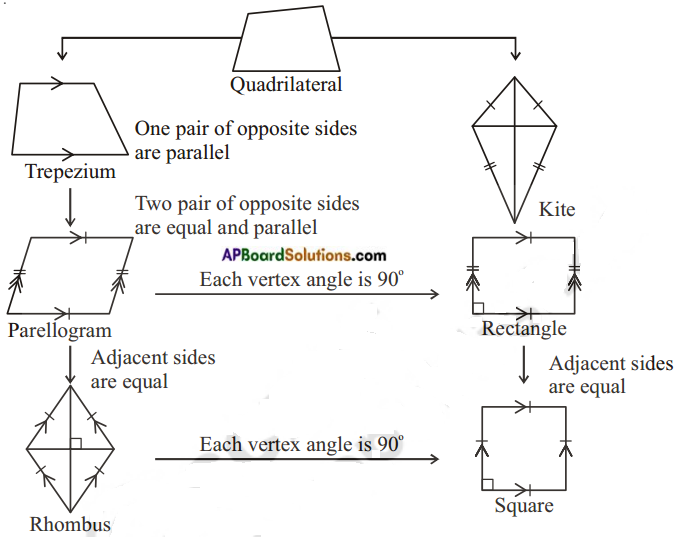Students can go through AP Board 8th Class Maths Notes Chapter 3 Construction of Quadrilaterals to understand and remember the concepts easily.

## AP State Board Syllabus 8th Class Maths Notes Chapter 3 Construction of Quadrilaterals

→ A closed four sided polygon is called a quadrilateral.

→ A quadrilateral has 4 sides, 4 vertices, 4 angles and 2 diagonals.→ The sum of the 4 angles of a quadrilateral is 360°.

 Type of a quadrilateral No. of individual measurements 1. Quadrilateral 5 2. Trapezium 4 3. Parallelogram 3 4. Rectangle 3 5. Rhombus 2 6. Square 1→ Five independent measurements are required to draw a unique quadrilateral.→ A quadrilateral can be constructed uniquely, if
a) The lengths of four sides and one angle are given
b) The lengths of four sides and one diagonal are given
c) The lengths of three sides and two diagonals are given
d) The lengths of two adjacent sides and three angles are given
e) The lengths of three sides and two included angles are given

→ The two special quadrilaterals, namely rhombus and square can be constructed when two diagonals are given.